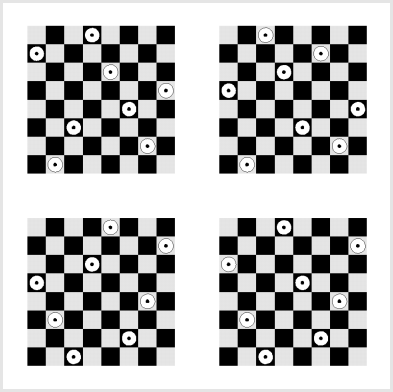## The n-Queens ProblemThe n-queens problem is the problem to compute how many ways n queens can be arranged on an (n x n) chess board so that no two queens attack each other.

Here I present a Mathematica notebook which contains implementations of several Mathematica functions from the book

I. Vardi, Computational Recreations in Mathematica, Addison-Wesley, 1991.

Furthermore, the notebook contains tools for printing graphics of solutions of the n-queens problem.